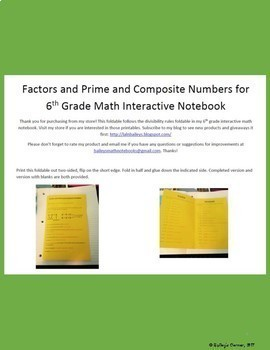# Unit 1 Bundle for 6th Grade Math Notebook: Fraction/Decimal Operations6th, Homeschool
Subjects
Standards
Resource Type
Formats Included
• Zip
Pages
93 pages
\$25.20
Bundle
List Price:
\$36.00
You Save:
\$10.80
\$25.20
Bundle
List Price:
\$36.00
You Save:
\$10.80
Total Pages
93 pages
Included
Teaching Duration
N/A
Report this Resource to TpT
Reported resources will be reviewed by our team. Report this resource to let us know if this resource violates TpT’s content guidelines.

### Standards

to see state-specific standards (only available in the US).
Interpret and compute quotients of fractions, and solve word problems involving division of fractions by fractions, e.g., by using visual fraction models and equations to represent the problem. For example, create a story context for (2/3) ÷ (3/4) and use a visual fraction model to show the quotient; use the relationship between multiplication and division to explain that (2/3) ÷ (3/4) = 8/9 because 3/4 of 8/9 is 2/3. (In general, (𝘢/𝘣) ÷ (𝘤/𝘥) = 𝘢𝘥/𝘣𝘤.) How much chocolate will each person get if 3 people share 1/2 lb of chocolate equally? How many 3/4-cup servings are in 2/3 of a cup of yogurt? How wide is a rectangular strip of land with length 3/4 mi and area 1/2 square mi?
Fluently divide multi-digit numbers using the standard algorithm.
Fluently add, subtract, multiply, and divide multi-digit decimals using the standard algorithm for each operation.
Find the greatest common factor of two whole numbers less than or equal to 100 and the least common multiple of two whole numbers less than or equal to 12. Use the distributive property to express a sum of two whole numbers 1–100 with a common factor as a multiple of a sum of two whole numbers with no common factor. For example, express 36 + 8 as 4 (9 + 2).